# Euclids geometry class 9 notes

Welcome to Class 9 Maths chapter 5 Euclids Geometry notes.The topics in this page are Euclids Geometry ,Axioms or Postulates and Euclids axioms along with examples, and questions and answers .This is according to CBSE and the NCERT textbook. If you like the study material, feel free to share the link as much as possible.

Table of Content

## Euclids Geometry

• The word 'geometry' comes form the Greek words 'geo', meaning the 'earth', and 'metrein', meaning 'to measure
• Euclid a Greek mathematician is called the Father of Geometry
• Euclid collected all the known work in his time and created Elements .He divided the 'Elements' into thirteen chapters, each called a book.
• Euclid defined around 23 items. 7 of them are mentioned below

Euclid's definition
1. A point is that which has no part
2. A line is breath less and has length only
3. The end of a line is points
4. A straight line is a line which lies evenly with the points on itself
5. A surface is that which has length and breath only
6. The edges of a surface are lines

The definitions of line, point, plane explained by Euclid is not accepted by the Mathematician. So these terms are taken as undefined

## Axioms or Postulates

Axioms or Postulates are assumptions which are obvious universal truths. They are not proved
Euclid axioms are the assumptions which are used throughout mathematics while Euclid Postulates are the assumptions which are specific to geometry

## Statements

A sentence which is either True or false but but not both is called the statement.

## Theorems

They are statements which are proved using axioms/postulates, definition, previously proved statement and deductive reasoning

## Corollary

A "Corollary" is a theorem that is usually considered an "easy consequence" of another theorem. What is or is not a corollary is entirely subjective

## Euclid axioms

1. Things which are equal to same things are equal to one another
a) If $x=z$, $y=z$ then $x=y$
b) if an area of a triangle equals the area of a rectangle and the area of the rectangle equals that of a square, then the area of the triangle also equals the area of the square
2. If equals are added to equals, the wholes are equal
1. $x=y$ => $x+z=y+z$

We can use Second axiom as below
2. $x -10 =100$
=> $x-10 +10 = 100 +10$
=> $x=110$
3. If equals are subtracted from equals, the remainders are equal
$x=y$ => $x-z=y-z$
4. Things which coincide with one another are equal to one another
This seems to say that if two things are identical (that is, they are the same), then they are equal. In other words, everything equals itself. It is the justification of the principle of superposition.
5. The whole is greater than the part
if a quantity P is part of quantity Q , then Q > P. or we can that there exists a third quantity R such that Q=P+R
6. Things which are double of the same things are equal to one another
7. Things which are halves of the same things are equal to one another
8. If first thing is greater than second and second is greater than third, then first is greater than third

## Euclids Postulates

Here are the Euclids Postulates
1. A straight line may be drawn from one point to another point. As per this postulate,there exists a unique line joining two points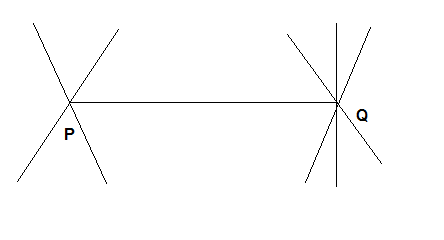2. A terminated line can be produced indefinitely. A terminated line or line segment is the segment of line joined by two separate point. As per this postulate, a line segment can be extended in in both direction to make a line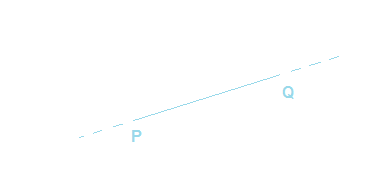3. A circle can be drawn with any centre and any radius. As per this postulate, we can draw circles with any centre and any radius. For example,we can draw a circle on line segment with centre at its end point and radius as the length of the line segments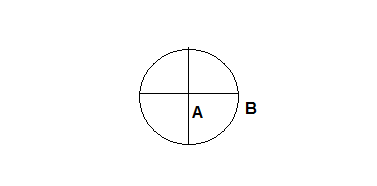4. All right angles are equal to one another
. A right angle is angle measuring $90^0$. As per this postulate ,all the right angles are equal and if we place one right angle over top of another right angle, they will coincide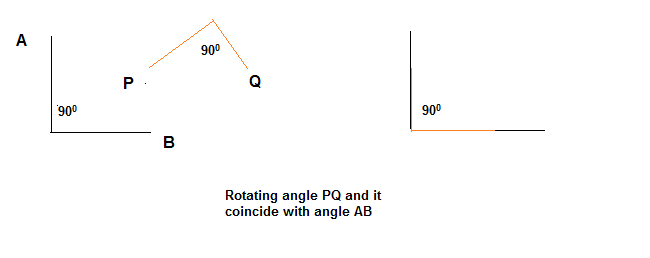5. If a straight line falling on two straight lines makes the interior angles on the same side of it taken together less than two right angles, then the straight lines if produced indefinitely meet on that side on which the angles are less than the two right angles

Here the line PQ in below figure falls on lines AB and CD such that the sum of the interior angles 1 and 2 is less than $180^0$ on the left side of PQ. Therefore, the lines AB and CD will eventually intersect on the left side of PQ.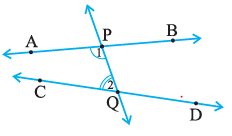6. ## Play fair Axiom

This is equivalent version of Euclid fifth law which states lines will not intersect when the angle is equal two right angle
For every line l and for every point P not lying on the line l, there exists a unique line m passing through P and Parallel to l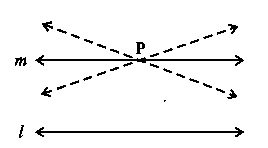## Some More important definition's

• Point: It is denoted by a single dot on the paper and it is represented by English alphabet. It has no length ,breadth and thickness
• Line: A line is straight and has no curves. It extends in both the direction and has no end point. We have infinite lines through a point while we will have only one line through two points
• Line Segment:If we take a portion of a line ,then it is called line segment and it has length and two end points. It can be measured
• Ray: If a line has one end point and it can extent in other direction,then it is called a ray
• Congruent segments: If two line segments are equal then they are called congruent

## Incident Axioms

1. A line contains infinitely many points
2. An infinite lines can be drawn through a point
3. If we have two given points,then there exists only one line which have both the points on it
4. Three or more points are collinear if they line on the same line
5. Two or more lines are concurrent lines if there is a point which lies on all of them
Crossword for Euclids Geometry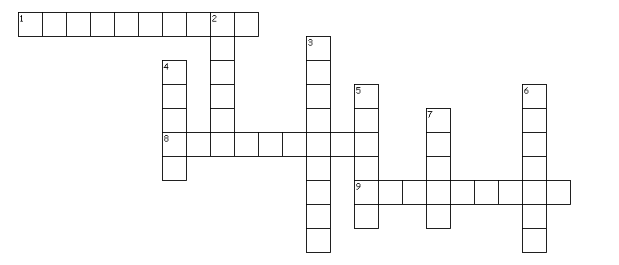Across
1. He was the student of Thales.
8. The terms specifically used for assumption in Geometry.
9. A _______ is a theorem that is usually considered an "easy consequence" of another theorem.
Down
2. Square and circular ____ were used for household rituals.
3. The instrument consists of nine interwoven isosceles triangles
4. Euclid was a teacher of mathematics in Alexandria in _______
5. Thales belongs to ______
6. They are the statements which are proved
7. The term used for the assumptions which are used throughout mathematics

### Quiz Time

Question 1 It is known that if $x + z = 100$ then $x + y + z = 100 + y$. The Euclid's axiom that illustrates this statement is ?
A. Ist
B. IInd
C. IIIrd
D. IVth
Question 2 Which of these is false
A. Things equal to the same thing are equal
B. Euclid's fourth axiom says that everything equals itself.
C. A point has no dimension
D. The statements that are proved are called axioms.
Question 3 Boundaries of solids are
A. Surfaces
B. curve
C. line
D. points
Question 4 Euclid divided his famous treatise "The Elements" into :
A. 9 chapters
B. 23 chapters
C. 10 chapters
D. 13 chapters
Question 5 The things which are double of the same thing are
A. half of others
B. double
C. equals
D. none of the above
Question 6 Euclid stated that all right angles are equal to each other in the form of ?
A. Axiom
B. definition
C. theorem
D. postulate

## Summary

Here is Euclids geometry class 9 notes Summary
• The postulates or axioms are the evident universal truths that serve as assumptions. They are not supported by evidence.
• Theorems are assertions that are supported by definitions, axioms, earlier assertions that have also been supported by evidence, and deductive reasoning.
• Postulate 1 : A straight line may be drawn from any one point to any other point.
• Postulate 2 : A terminated line can be produced indefinitely.
• Postulate 3 : A circle can be drawn with any centre and any radius.
• Postulate 4 : All right angles are equal to one another.
• Postulate 5 : If a straight line falling on two straight lines makes the interior angles on the same side of it taken together less than two right angles, then the two straight lines, if produced indefinitely, meet on that side on which the sum of angles is less than two right angles

• Notes
• NCERT Solutions
• Assignments
• Revision sheet